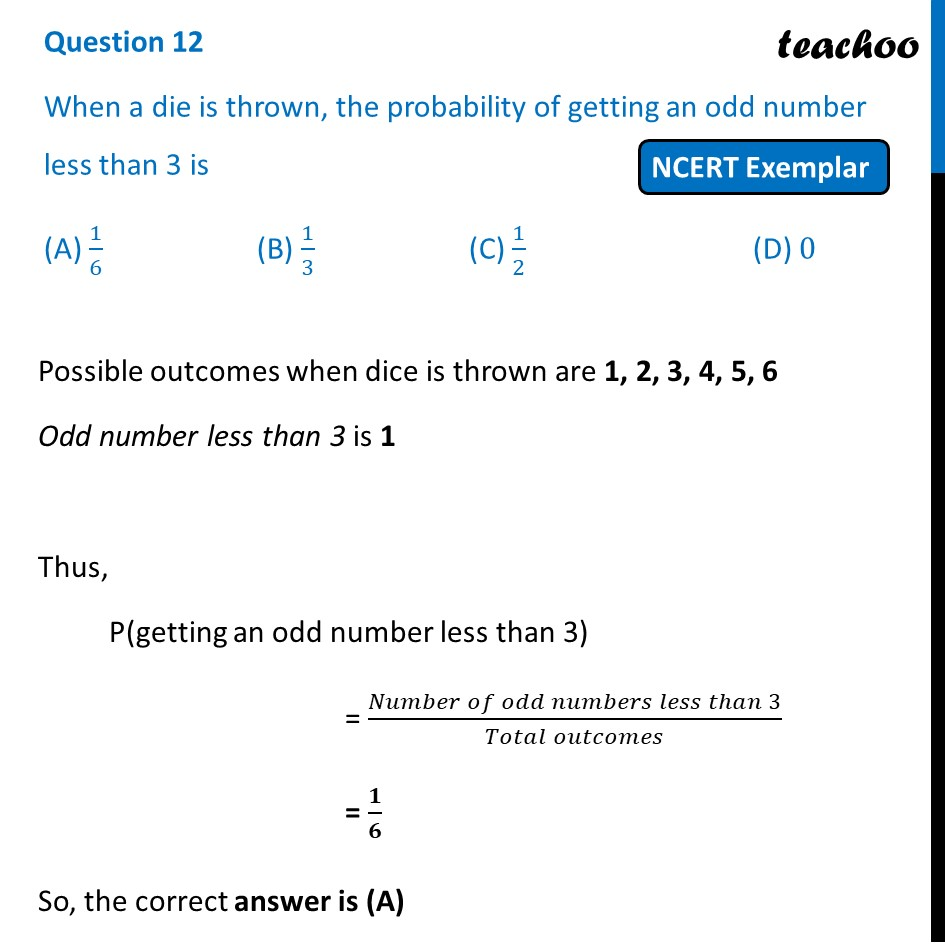## (A) 1/6  (B) 1/3  (C) 1/2    (D) 01. Chapter 15 Class 10 Probability (Term 1)
2. Serial order wise
3. NCERT Exemplar - MCQ

Transcript

Question 12 When a die is thrown, the probability of getting an odd number less than 3 is (A) 1/6 (B) 1/3 (C) 1/2 (D) 0 Possible outcomes when dice is thrown are 1, 2, 3, 4, 5, 6 Odd number less than 3 is 1 Thus, P(getting an odd number less than 3) = (𝑁𝑢𝑚𝑏𝑒𝑟 𝑜𝑓 𝑜𝑑𝑑 𝑛𝑢𝑚𝑏𝑒𝑟𝑠 𝑙𝑒𝑠𝑠 𝑡ℎ𝑎𝑛 3)/(𝑇𝑜𝑡𝑎𝑙 𝑜𝑢𝑡𝑐𝑜𝑚𝑒𝑠) = 𝟏/𝟔 So, the correct answer is (A)